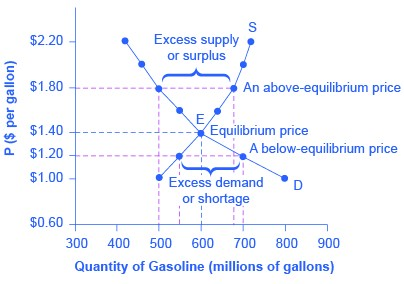### Concepts of demand and supply – Determinants of equilibrium price, quantity and point

Economics SSS1 First Term

WEEK 6

Concepts of demand and supply – Determinants of equilibrium price, quantity and point

Performance Objectives

Student should be able to:

1. Identify and explain the determinants of equilibrium price, quantity and point with simple applications.

Content

The equilibrium price is the market price where the quantity of goods supplied is equal to the quantity of goods demanded. This is the point at which the demand and supply curves in the market intersect. To determine the equilibrium price, you have to figure out at what price the demand and supply curves intersect.

Figure 1 illustrates the interaction of demand and supply in the market for gasoline.Figure 1. Demand and Supply for Gasoline.

The demand curve (D) and the supply curve (S).... View More